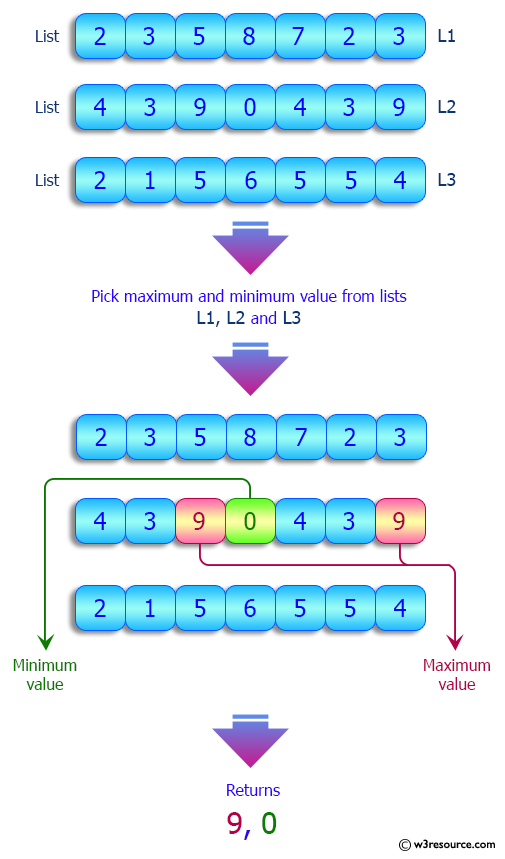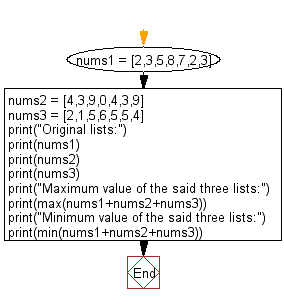﻿ Python: Maximum and minimum value of the three given lists - w3resource# Python: Maximum and minimum value of the three given lists

## Python List: Exercise - 191 with Solution

Write a Python program to find the maximum and minimum value of the three given lists.

Sample Solution:

Python Code:

``````nums1 = [2,3,5,8,7,2,3]
nums2 = [4,3,9,0,4,3,9]
nums3 = [2,1,5,6,5,5,4]
print("Original lists:")
print(nums1)
print(nums2)
print(nums3)
print("Maximum value of the said three lists:")
print(max(nums1+nums2+nums3))
print("Minimum value of the said three lists:")
print(min(nums1+nums2+nums3))
```
```

Sample Output:

```Original lists:
[2, 3, 5, 8, 7, 2, 3]
[4, 3, 9, 0, 4, 3, 9]
[2, 1, 5, 6, 5, 5, 4]
Maximum value of the said three lists:
9
Minimum value of the said three lists:
0
```

Pictorial Presentation:Flowchart:## Visualize Python code execution:

The following tool visualize what the computer is doing step-by-step as it executes the said program:

Python Code Editor:

Have another way to solve this solution? Contribute your code (and comments) through Disqus.

What is the difficulty level of this exercise?

Test your Python skills with w3resource's quiz

﻿

## Python: Tips of the Day

Floor Division:

When we speak of division we normally mean (/) float division operator, this will give a precise result in float format with decimals.

For a rounded integer result there is (//) floor division operator in Python. Floor division will only give integer results that are round numbers.

```print(1000 // 300)
print(1000 / 300)```

Output:

```3
3.3333333333333335```